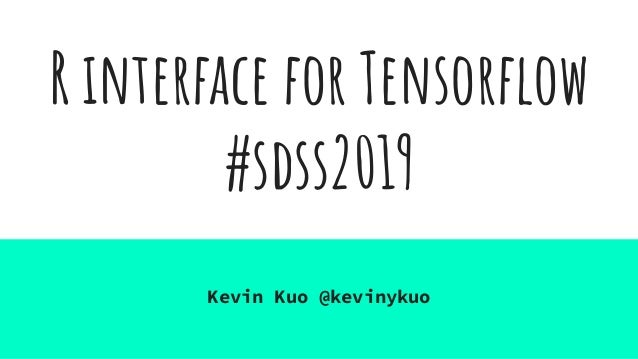Successfully reported this slideshow.Upcoming SlideShare
×

# R Interface for TensorFlow

17 views

Published on

We provide an overview of the tools that enable deep learning in R, including packages such as tensorflown keras, and tfestimators. Demos are included to show the API. We also discuss the latest features.

Published in: Technology
• Full Name
Comment goes here.

Are you sure you want to Yes No• Be the first to comment

• Be the first to like this

### R Interface for TensorFlow

1. 1. R interface for Tensorﬂow #sdss2019 Kevin Kuo @kevinykuo
2. 2. Artiﬁcial intelligence?
3. 3. Artiﬁcial Intelligence? Getty Images
4. 4. Getty Images Artiﬁcial Intelligence?
5. 5. Rule #1 of AI:
6. 6. Rule #1 of AI: Someone talk to you about AI with a straight face? They tryna hustle ya.
7. 7. ELI5 TensorFlow?
8. 8. ELI5 a stats undergrad TensorFlow
9. 9. What’s a tensor?
10. 10. What’s a tensor? What’s ﬂowing?
11. 11. Tensors & Ops Tensors are just multidimensional arrays
12. 12. Tensors & Ops Tensors are just multidimensional arrays You apply Ops (transformations ) to Tensors to get more Tensors
13. 13. Deep Learning? Neural networks?
14. 14. Find a function F such that Y ≈ f(x)
15. 15. Find a function F such that Y ≈ f(x) (at least some of the time)
16. 16. Find a function F such that Y ≈ f(x) (at least some of the time, hopefully)
17. 17. Really just straightforward matrix algebra
18. 18. Resources
19. 19. tensorﬂow https://www.tensorflow.org/guide/extend/ar chitecture
20. 20. tensorﬂow tf.keras
21. 21. tensorﬂow tf.keras library(keras)
22. 22. library(tfdatasets) library(tfprobability) library(tensorflow)
23. 23. GLM is a neural net! We’re doing AI!
24. 24. Proof (by example).
25. 25. library(keras) library(tfprobability) model <- keras_model_sequential() %>% layer_dense(units = 1, input_shape = 1, name = "parameters") %>% layer_lambda(f = k_exp) %>% layer_distribution_lambda(tfd_poisson)
26. 26. library(keras) library(tfprobability) model <- keras_model_sequential() %>% layer_dense(units = 1, input_shape = 1, name = "parameters") %>% layer_lambda(f = k_exp) %>% layer_distribution_lambda(tfd_poisson) neg_loglik <- function(y_true, y_pred) { - tfd_log_prob(y_pred, y_true) } model %>% compile(optimizer = optimizer_sgd(lr = 0.1), loss = neg_loglik)
27. 27. model <- keras_model_sequential() %>% layer_dense(units = 1, input_shape = 1, name = "parameters") %>% layer_lambda(f = k_exp) %>% layer_distribution_lambda(tfd_poisson) neg_loglik <- function(y_true, y_pred) { - tfd_log_prob(y_pred, y_true) } model %>% compile(optimizer = optimizer_sgd(lr = 0.1), loss = neg_loglik) model %>% fit(x, y, epochs = 15)
28. 28. > parameters <- model %>% + get_layer("parameters") %>% + (function(x) x\$get_weights()) > parameters [] [,1] [1,] 1.985788 []  1.090006
29. 29. > glm(y ~ x, family = poisson()) Call: glm(formula = y ~ x, family = poisson()) Coefficients: (Intercept) x 1.090 1.986 Degrees of Freedom: 99 Total (i.e. Null); 98 Residual Null Deviance: 359 Residual Deviance: 90.1 AIC: 484.2
30. 30. > parameters <- model %>% + get_layer("parameters") %>% + (function(x) x\$get_weights()) > parameters [] [,1] [1,] 1.985788 []  1.090006
31. 31. model <- keras_model_sequential() %>% layer_dense(units = 8675309, input_shape = 1, name = "parameters") %>% layer_dense(units = 8675309) %>% layer_lambda(f = k_exp) %>% layer_distribution_lambda(tfd_poisson) NOW YOU HAVE A DEEP NEURAL NET
32. 32. model <- keras_model_sequential() %>% layer_dense(units = 8675309, input_shape = 1, name = "parameters") %>% layer_dense_variational( units = 1, make_posterior_fn = posterior_mean_field, make_prior_fn = prior_trainable, kl_weight = 1 / n_rows, activation = "linear" ) %>% layer_lambda(f = k_exp) %>% layer_distribution_lambda(tfd_poisson) NOW YOUR MODEL IS A RANDOM VARIABLE ZOMG
33. 33. model <- keras_model_sequential() %>% layer_dense(units = 8675309, input_shape = 1, name = "parameters") %>% layer_dense_variational( units = 2, make_posterior_fn = posterior_mean_field, make_prior_fn = prior_trainable, kl_weight = 1 / n_rows, activation = "linear" ) %>% layer_distribution_lambda(function(t) { tfd_normal(t[,1], k_softplus(t[,2])) }) HETEROSCEDASTIC ERRORS OMGWTFBBQ
34. 34. model <- keras_model_sequential() %>% ____ %>% ____ %>% ____ model %>% compile(optimizer = ____, loss = ____) THE POSSIBILITIES ARE ENDLESS
35. 35. model <- keras_model_sequential() %>% ____ %>% ____ %>% ____ model %>% compile(optimizer = ____, loss = ____) THE POSSIBILITIES ARE ENDLESS
36. 36. Why should I give a ___ about deep learning?
37. 37. Enabling new applications?
38. 38. 1.123% Percentage of data scientists who work with image data on the job
39. 39. Things neural nets can help with - Images (yeah, sometimes we get them) - Natural language / Time series - High dimensional categorical predictors - Multi-task learning, i.e. when we want to predict multiple outputs with a single model - Combining different types of inputs into the same model - Making predictions on devices without docker containers (e.g. your phone)
40. 40. Resources ● https://tensorflow.rstudio.com/ ● https://keras.rstudio.com/ ● https://blogs.rstudio.com/tensorflow/ ● https://github.com/rstudio/tfprobability